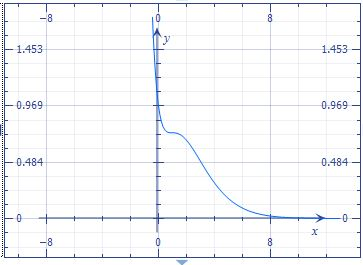# Let f be the function defined by f(x) = (x2 + 1)e6{-x} for -4 \leq x \leq 4. a) For what value of...

## Question:

Let {eq}\displaystyle f {/eq} be the function defined by {eq}\displaystyle f(x) = (x^2 + 1)e^{-x} {/eq} for {eq}\displaystyle -4 \leq x \leq 4. {/eq}

a) For what value of {eq}\displaystyle x {/eq} does {eq}\displaystyle f {/eq} reach its absolute maximum? Justify your answer. (You may use your calculator to evaluate the {eq}\displaystyle x {/eq} values in {eq}\displaystyle f(x). {/eq})

b) Find the {eq}\displaystyle x {/eq} coordinates of all points of inflection of {eq}\displaystyle f {/eq}. Justify your answer.

## Inflection point and Absolute maximum

We find the inflection points of a function with the second derivative. In function there is an inflection point when it changes concavity, it can stop being concave up and begins to be concave down at the inflection point.

Become a Study.com member to unlock this answer! Create your account

We have the function

{eq}f(x)= (x^2 + 1)e^{-x} \\ {/eq}

Graph the functionDifferentiating the function

{eq}f'(x)=-{{\rm e}^{-x}} \left(...